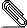# Re: [HACKERS] PoC: Partial sort

`On Tue, Aug 19, 2014 at 2:02 PM, David Rowley <dgrowle...@gmail.com> wrote:`
```
> Here's a few notes from reading over the code:
>
> * pathkeys.c
>
>   EquivalenceMember *member = (EquivalenceMember *)
>
> occurs in costsize.c
>

Fixed.

> * pathkeys.c
>
> The following fragment:
>
> n = pathkeys_common(root->query_pathkeys, pathkeys);
>
> if (n != 0)
> {
> /* It's useful ... or at least the first N keys are */
>  return n;
> }
>
> return 0; /* path ordering not useful */
> }
>
>
> /* return the number of path keys in common, or 0 if there are none */
>  return pathkeys_common(root->query_pathkeys, pathkeys);
>

Fixed.

> * execnodes.h
>
> In struct SortState, some new fields don't have a comment.
>

Fixed.

> I've also thrown a few different workloads at the patch and I'm very
> impressed with most of the results. Especially when LIMIT is used, however
> I've found a regression case which I thought I should highlight, but for
> now I can't quite see what could be done to fix it.
>
> create table a (x int not null, y int not null);
> insert into a select x.x,y.y from generate_series(1,1000000) x(x) cross
> join generate_series(1,10) y(y);
>
> Patched:
> explain analyze select x,y from a where x+0=1 order by x,y limit 10;
>                                                              QUERY PLAN
>
> ------------------------------------------------------------------------------------------------------------------------------------
>  Limit  (cost=92.42..163.21 rows=10 width=8) (actual
> time=6239.426..6239.429 rows=10 loops=1)
>    ->  Partial sort  (cost=92.42..354064.37 rows=50000 width=8) (actual
> time=6239.406..6239.407 rows=10 loops=1)
>          Sort Key: x, y
>          Presorted Key: x
>          Sort Method: quicksort  Memory: 25kB
>          ->  Index Scan using a_x_idx on a  (cost=0.44..353939.13
> rows=50000 width=8) (actual time=0.059..6239.319 rows=10 loops=1)
>                Filter: ((x + 0) = 1)
>                Rows Removed by Filter: 9999990
>  Planning time: 0.212 ms
>  Execution time: 6239.505 ms
> (10 rows)
>
>
> Time: 6241.220 ms
>
> Unpatched:
> explain analyze select x,y from a where x+0=1 order by x,y limit 10;
>                                                      QUERY PLAN
>
> --------------------------------------------------------------------------------------------------------------------
>  Limit  (cost=195328.26..195328.28 rows=10 width=8) (actual
> time=3077.759..3077.761 rows=10 loops=1)
>    ->  Sort  (cost=195328.26..195453.26 rows=50000 width=8) (actual
> time=3077.757..3077.757 rows=10 loops=1)
>          Sort Key: x, y
>          Sort Method: quicksort  Memory: 25kB
>          ->  Seq Scan on a  (cost=0.00..194247.77 rows=50000 width=8)
> (actual time=0.018..3077.705 rows=10 loops=1)
>                Filter: ((x + 0) = 1)
>                Rows Removed by Filter: 9999990
>  Planning time: 0.510 ms
>  Execution time: 3077.837 ms
> (9 rows)
>
>
> Time: 3080.201 ms
>
> As you can see, the patched version performs an index scan in order to get
> the partially sorted results, but it does end up quite a bit slower than
> the seqscan/sort that the unpatched master performs. I'm not quite sure how
> realistic the x+0 = 1 WHERE clause is, but perhaps the same would happen if
> something like x+y = 1 was performed too.... After a bit more analysis on
> this, I see that if I change the 50k estimate to 10 in the debugger that
> the num_groups is properly estimated at 1 and it then performs the seq scan
> instead. So it looks like the costings of the patch are not to blame here.
> (The 50k row estimate comes from rel tuples  / DEFAULT_NUM_DISTINCT)
>

Yes, the error comes from assumption of 50k row estimate. I've checked
similar example when estimate is fine.

create table b as (select x.x,y.y,x.x z from generate_series(1,1000000)
x(x) cross join generate_series(1,10) y(y));
create index b_x_idx on b(x);
analyze b;

There is column z which is both not in index and not in "order by" clause.
If we replace "x+0=1" with "z=1" optimizer didn't decide to use partial
sort.

explain analyze select x,y,z from b where z=1 order by x,y limit 10;
QUERY PLAN
──────────────────────────────────────────────────────────────────────────────────────────────────────────────────
Limit  (cost=179056.59..179056.61 rows=10 width=12) (actual
time=1072.498..1072.500 rows=10 loops=1)
->  Sort  (cost=179056.59..179056.63 rows=18 width=12) (actual
time=1072.495..1072.495 rows=10 loops=1)
Sort Key: x, y
Sort Method: quicksort  Memory: 25kB
->  Seq Scan on b  (cost=0.00..179056.21 rows=18 width=12) (actual
time=0.020..1072.454 rows=10 loops=1)
Filter: (z = 1)
Rows Removed by Filter: 9999990
Planning time: 0.501 ms
Execution time: 1072.555 ms
(9 rows)

If we event force optimizer to use partial sort then cost estimation will
be fine.

set enable_seqscan = off;
explain analyze select x,y,z from b where z=1 order by x,y limit 10;
QUERY PLAN
──────────────────────────────────────────────────────────────────────────────────────────────────────────────────────────────────
Limit  (cost=169374.43..263471.04 rows=10 width=12) (actual
time=2237.082..2237.083 rows=10 loops=1)
->  Partial sort  (cost=169374.43..338748.34 rows=18 width=12) (actual
time=2237.082..2237.083 rows=10 loops=1)
Sort Key: x, y
Presorted Key: x
Sort Method: quicksort  Memory: 25kB
->  Index Scan using b_x_idx on b  (cost=0.43..338748.13 rows=18
width=12) (actual time=0.047..2237.062 rows=10 loops=1)
Filter: (z = 1)
Rows Removed by Filter: 9999990
Planning time: 0.089 ms
Execution time: 2237.133 ms
(10 rows)

AFAICS wrong selectivity estimations are general problem which cause
optimizer failures. But in your example "x+y=1" if expression index on
"x+y" would exist then statistics over "x+y" will be collected. So, in case
of expression index estimation will be fine.

------
With best regards,
Alexander Korotkov.
```partial-sort-basic-2.patch
Description: Binary datapartial-sort-merge-2.patch
Description: Binary data

```--
Sent via pgsql-hackers mailing list (pgsql-hackers@postgresql.org)
To make changes to your subscription:
http://www.postgresql.org/mailpref/pgsql-hackers
```